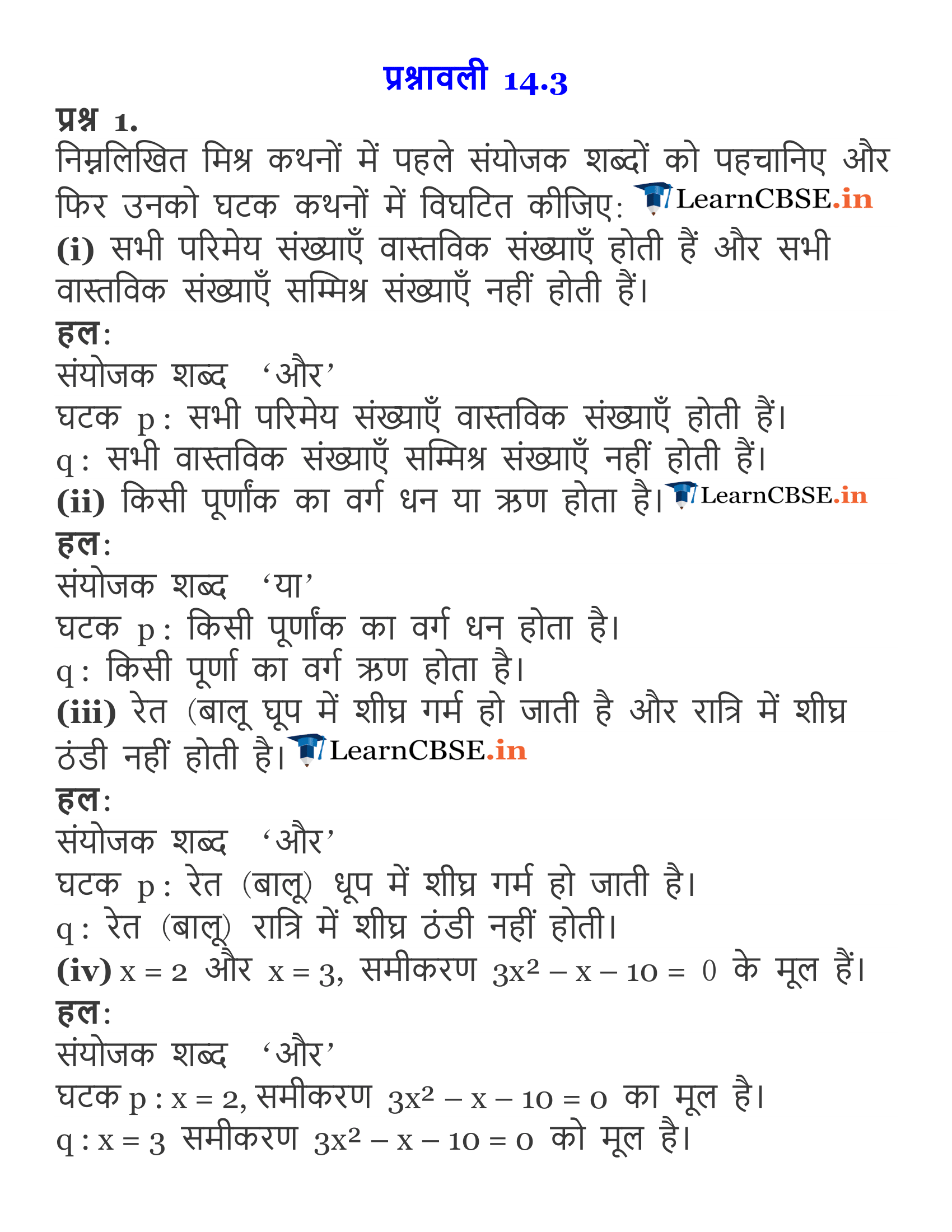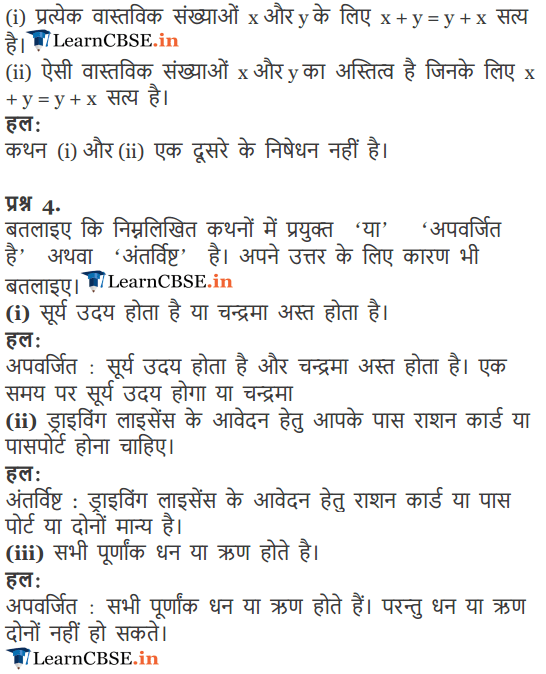Get Free NCERT Solutions for Class 11 Maths Chapter 14 Mathematical Reasoning Ex 14.3 PDF in Hindi and English Medium. Mathematical Reasoning Class 11 Maths NCERT Solutions are extremely helpful while doing your homework. Mathematical Reasoning Ex 14.3 Class 11 Maths NCERT Solutions were prepared by Experienced LearnCBSE.in Teachers. Detailed answers of all the questions in Chapter 14 Class 11 Mathematical Reasoning Ex 14.3 provided in NCERT Textbook.

Free download NCERT Solutions for Class 11 Maths Chapter 14 Mathematical Reasoning Ex 14.3 PDF in Hindi Medium as well as in English Medium for CBSE, Uttarakhand, Bihar, MP Board, Gujarat Board, BIE, Intermediate and UP Board students, who are using NCERT Books based on updated CBSE Syllabus for the session 2019-20.

## NCERT Solutions for Class 11 Maths Chapter 14 Ex 14.3

### NCERT Solutions for Class 11 Maths Chapter 14 Exercise.14.3

Question 1.
For each of the following compound statements first, identify the connecting words and then break it into component statements.
(i) All rational numbers are real and all real numbers are not complex.
(ii) Square of an integer is positive or negative.
(iii) The sand heats up quickly in the Sun and does not cool down fast at night.
(iv) x = 2 and x = 3 are the roots of the equation 3x2 – x – 10 = 0.
Solution:
(i) The compound statement has the connecting word ‘and’. Component statements are
p: All rational numbers are real.
q: All real numbers are not complex.

(ii) The compound statement has the connecting word ‘or’. Component statements are:
p: Square of an integer is positive.
q: Square of an integer is negative.

(iii) The compound statement has the connecting word ‘and’. Component statements are:
p: The sand heats up quickly in the sun.
q: The sand does not cool down fast at night.

(iv) The compound statement has the connecting word ‘and’. Component statements are:
p: x- 2 is a root of the equation 3x2 – x – 10 = 0.
q: x = 3 is a root of the equation 3x2 – x – 10 = 0.

Question 2.
Identify the quantifier in the following statements and write the negation of the statements.
(i) There exists a number which is equal to its square.
(ii) For every real number x, x is less than x + 1.
(iii) There exists a capital for every state in India.
Solution:
(i) Here the quantifier is ‘there exists’.
The negation of statement is: There does not exist a number which is equal to its square.
(ii) Here the quantifier is ‘for every’
The negation of statement is: For at least one real number x, x is not less than x + 1.
(iii) Here the quantifier is ‘there exists’
The negation of statement is: There exists a state in India which does not have a capital.

Question 3.
Check whether the following pair of statements is negation of each other. Give reasons for your answer.
(i) x + y = y + x is true for every real numbers x and y.
(ii) There exists real numbers x and y for which x + y = y + x.
Solution:
Let p: x + y = y + x is true for every real numbers x and y.
q: There exists real numbers x and y for which
x+y=y + x.
Now, ~p: There exists real numbers x and y for which x + y ≠ y + x.
Thus, ~p ≠ q.

Question 4.
State whether the “Or” used in the following statements is “exclusive” or “inclusive”. Give reasons for your answer.
(i) Sunrises or Moon sets.
(ii) To apply for a driving license, you should have a ration card or a passport.
(iii) All integers are positive or negative.
Solution:
(i) This statement makes use of exclusive “or”. Since when sun rises, moon does not set during day-time.
(ii) This statement makes use of inclusive ‘or’. Since you can apply for a driving license even if you have a ration card as well as a passport.
(iii) This statement makes use of exclusive ‘or’. Since a integer is either positive or negative, it cannot be both.

### NCERT Solutions for Class 11 Maths Chapter 14 Mathematical Reasoning (गणितीय विवेचन) Hindi Medium Ex 14.3